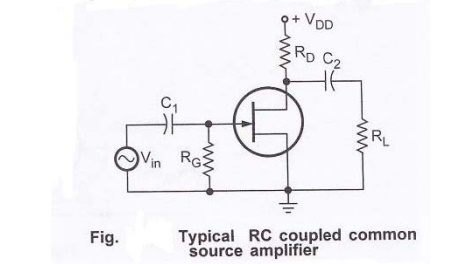Home | | Electronic Circuits I | Low frequency analysis of FET amplifier

# Low frequency analysis of FET amplifier

From above figure, it has two RC networks that affect its gain as the frequency is reduced below midrange.

Low frequency analysis of FET amplifier:From above figure, it has two RC networks that affect its gain as the frequency is reduced below midrange. These are,

1.     RC network formed by the input coupling capacitor C1 and input impedance of the amplifier.

2.     RC network formed by the output coupling capacitor and the output impedance looking in at the drain.

`Input RC network:

Lower critical frequency of this network is given as,The value of Rin(gate) can be determined from the data sheet as follows:The phase shift in low frequency input RC circuit is θ = tan-1 (XC1 / Rin )

Output RC network:

Lower critical frequency of this network is given as,The phase shift in low frequency output RC circuit is θ = tan-1 (XC2 / RD + RL)

Study Material, Lecturing Notes, Assignment, Reference, Wiki description explanation, brief detail
Electronic Circuits : Frequency Analysis of BJT and MOSFET Amplifiers : Low frequency analysis of FET amplifier |